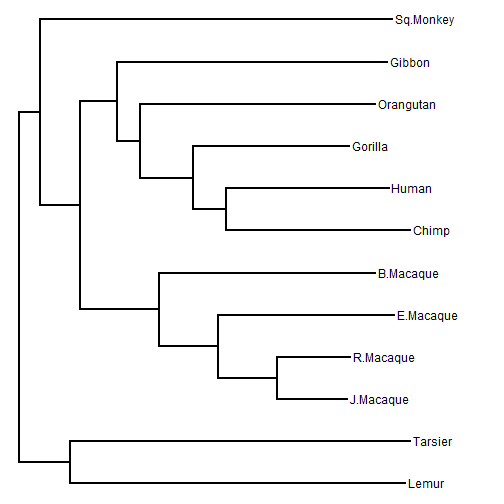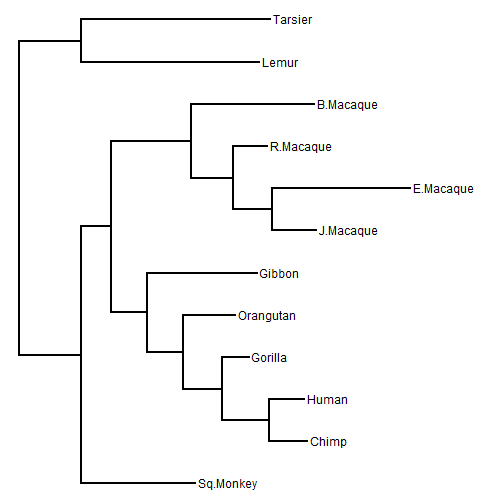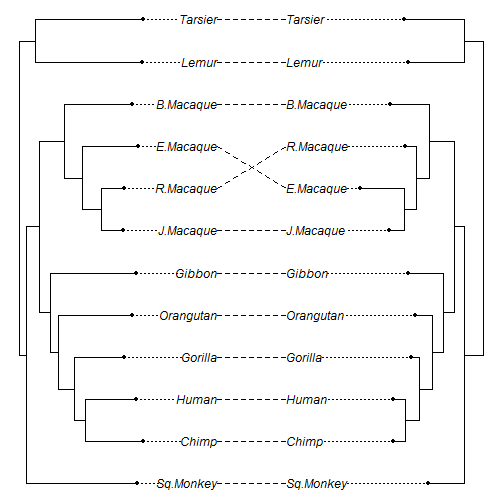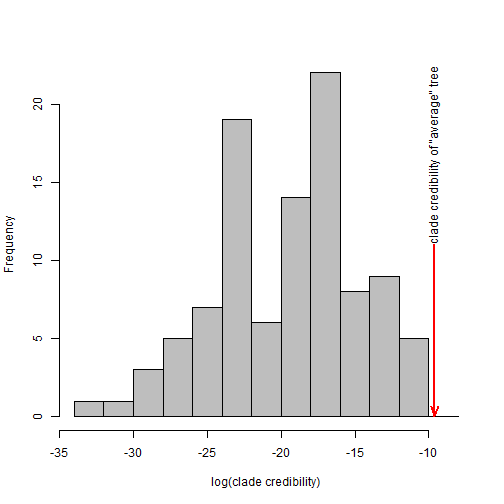## Sunday, April 10, 2016

### Average trees and maximum clade credibility trees

I have posted a couple of times on so-called average trees. This is the practice of identifying the phylogeny with the minimum sum of squares distances to the trees in a set. This is something I got into primarily because I was interested in summarizing distributions of trees from comparative methods such as stochastic character mapping.

Though I stumbled into it by accident, I'm discovering that there is a literature on average trees - although in phylogenetic biology, at least, it doesn't seem to be particularly large (compared to that on the different task of computing consensus trees). One significant contribution is an article dating to 1997 by Lapointe & Cucumel entitled “The Average Consensus Procedure: Combination of Weighted Trees Containing Identical or Overlapping Sets of Taxa.” This paper describes a procedure that I independently discovered (20 years later) and have implemented in the phytools function `ls.consensus`.

According to this consensus method, the 1 through n for n trees patristic distance matrices, Di are computed and averaged to produce D, then a tree & branch lengths are identified in which the sum of squares difference between D and the patristic distance matrix of the consensus tree, Dconsensus is minimized.

Here is a quick demo using the sample of really bad primate trees from before:

``````library(phytools)
library(phangorn)
ls.con<-ls.consensus(trees)
``````
``````## Best Q = 0.0052509716725781
``````
``````## Solution found after 1 set of nearest neighbor interchanges.
``````
``````plotTree(ls.con<-midpoint(ls.con))
``````Now, average trees have been criticized - not least because (evidently) they are not guaranteed to have the Pareto property, meaning that it is not guaranteed that every bipartition present in the consensus can be found in at least one of the input trees!

However, in this simple case I thought it might be interesting to see how our average tree by this method compared to, say, a maximum clade credibility tree from the same input set. Note that this is not a maximum clade credibility from a Bayesian posterior distribution - however these trees do come from a probability distribution with expectation equal to the true tree and broad variance, so this could be equivalent to a sample from the posterior distribution under some circumstances, such as when there is a lot of uncertainty regarding phylogenetic relationships in the data.

We can compute the maximum clade credibility tree using phangorn - however to do this one will have to install the latest phangorn version from GitHub.

``````mcc<-maxCladeCred(trees,rooted=FALSE)
nn<-fastMRCA(mcc,"Lemur","Tarsier")
plotTree(mcc<-reroot(mcc,nn,mcc\$edge.length[which(mcc\$edge[,2]==nn)]/2))
``````Topologically this tree is a pretty good tree, but it is not the correct tree.

(To see how it is wrong, we can do this:

``````obj<-cophylo(ls.con,mcc)
``````
``````## Rotating nodes to optimize matching...
## Done.
``````
``````plot(obj)
``````Cool, right?)

Something that we usually cannot do is compute the clade credibility of a tree not in the set that we have drawn from our (posterior) probability distribution. However, here we have a tree who's credibility is of interest, and so we can compute its clade credibility* simply by tacking it on to the end of our `"multiPhylo"` object. (*Note that this is not precisely the clade credibility because we have included the clades from our reference tree in the calculation of the clade frequencies - but it is pretty close.)

``````obj<-list(unroot(ls.con))
obj<-c(trees,obj)
obj<-hist(cc[1:100],breaks=seq(-34,-8,by=2),col="gray",main="",
arrows(x0=cc,y0=0.5*max(obj\$counts),x1=cc,y1=0,col="red",
lwd=2,length=0.15,angle=20)
text(x=cc,y=0.5*max(obj\$counts),
``````So that means the average tree has a higher probability (credibility) than any tree in the set. Interesting.

One could reasonably point out that if we'd merely sampled more trees we would have surely sampled the tree with highest credibility, and then we would have found it to have the same credibility as our LS consensus tree. That's probably true in this case - but, remember, the number of trees for many taxa is vast and so it may not be the case - depending on the shape of our posterior density - that we sample the highest probability topology in our MCMC. (I'm not saying that we don't - just that it cannot be assumed to be guaranteed.)

1.I'm following this series of posts with great interest, thanks for sharing! I assume you are already familiar with this paper, but anyway since it is on my list on favorites:

http://sysbio.oxfordjournals.org/content/60/4/528

They talk about Bayesian estimators that minimize the risk under several distances.

1.Thanks Leonardo!

2.This comment has been removed by the author.

3.Hi Liam. I used this function to calculate an MCCT but the tree I got does not have support values from the branches. Any ideas to fix this? Gracias!!

Note: due to the very large amount of spam, all comments are now automatically submitted for moderation.